## Web Scraping In A Machine Learning Model :

Data analysis is a long process that requires data pre-processing as a part of it. In the case of machine learning and other statistical theories and projects, it is required that the valuable data is kept and the garbage data is thrown out.

The thing is that the data collection techniques are often loosely-knit and hence this results in certain inaccurate data or inconsistent data sets or many out of range of garbage values.

It is necessary to eliminate such data because it will cause hindrance in the use of the data and this can be done with the help of web scraping and this is where we come into the picture.

We, at Scrapingpass.com, are there to solve all your queries revolving around web scraping.

The thing is that scraping can be a complicated task but, with the help of our ‘Scraping Bots’, it becomes easy for any user to scrape any kind of data.

The coding used in our methodology is efficient and implemented to perfection. We have developed bots to scrape data from Amazon.com and it isn’t easy to create code every now and then.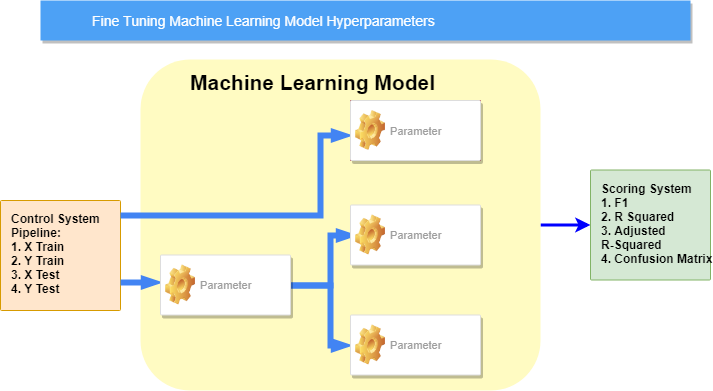KDnuggets

## Understanding The Relation Between Data Preprocessing and Web Scraping:

• Many times analysis of the user data requires data preprocessing. This means that the data is analyzed in a thorough manner in order to find inconsistencies.
• These inconsistencies depend upon the methods that are implemented and other statistical projects through which the results have occurred.
• Data preprocessing is quite complex. While it doesn’t mean that there is only one person that has to manage entire data and preprocess the whole data set by himself but, it will be way easier if he does it by himself.
• This is because when many people are involved, it is certain that the workload will lessen on each person but each person has a different approach towards different statistical methods.
• This means that with each person having a different mindset there will be a difference of opinions and perspectives for each of the datasets that they are giving.

If we jump to the definition of data preprocessing it is simple.

It can be said the data preprocessing is the simple act of removal of unnecessary or garbage data from the whole data set. The data set can include various things like the description of the data itself or the subject information for how the statistical variables were put or the description of the conditions under which they were put.

Thus, it is necessary to remove any information that is not worthy enough to be in the analysis of the whole data set.

Below we will be discussing how scraping can be used to collect data from the web pages and how they can be put into a CSV file format and stored on the local machine.

In machine learning, it can be said that there are about 7 of the most important data preprocessing steps in machine learning :

## The Seven Steps are :

1. Collection of the Data Set.
2. Importing all the data and the libraries that are necessary.
3. The dataset is then divided into two parts: independent and dependent variables
4. The missing values are handled.
5. Categorical values are checked.
6. Thereafter, splitting of data set takes places
7. Scaling of the featuresHir Infotech

But, this wouldn’t be the best guide if there’s no explanation, right? Let’s get to the details.

### 1. Collection of the Data Set :

Web scraping is simply the extraction of data from various web pages and websites across different servers in order to gather all the information or any selected information from the website.

Usually, the data that is mostly not available on the main page is accessed through a link that is posted on the main page is scraped. One of the most used methods for web scraping is by making use of the Python programming language.

• While web scraping and Pandas both have the same approach towards gathering the data, there are a few minute differences that can be seen like requests taking longer time because they need to compile many files in order to extract data from the web page.
• However, the point of difference between Pandas and request is that the former allows a number of different ways in which the required data can be taken whereas requests also allow the same but through the direct use of the website URL.
• The thing is that in requests there is no restriction as to the variety of URLs although requests are better suited to bigger web pages.
• Request are slower because of the increase in complexity.

This is the disadvantage that both methods have but it is something that any user should expect while he or she is trying to use modern complex programming languages when talking in a general sense.

But, if the goal of the user is to extract data through URLs from web pages across various servers then the difference that these two methods have in terms of speed is quite acceptable.

Therefore, here are a few tools along with libraries that can be used :

1. BeautifulSoup is a great tool for scraping information via HTML and XML pages.
2. Requests are responsible for sending HTTP requests with ease.
3. Fast, expressive, and flexible data structures are provided by Pandas.

### 2. Importing all the data and the libraries that are necessary :

Here are 3 of the most important libraries that will be used in the method of data preprocessing :

• For array computation in Python programming language, the fundamental package is Numpy. Insertion of any kind of mathematical operation or addition of multidimensional arrays with large values or matrices in a code can be done with the help of this library.
• Matplotlib.pyplot can be useful in the plotting of different types of charts into the code and is a 2D Python library.
• A powerful and extremely useful tool that can be used for the analysis of data along with the statistical reading of the data is Pandas. It is very helpful in the case of time series analysis too. Importation and management of data sets are also quite important in this case.

### 3. The dataset is then divided into two parts: independent and dependent variables :

In the case of Machine Learning platforms and models, it is quite imperative to import the data set by the extraction of the independent and dependent variables, and hence, it is mandatory to perform this step to continue with the web scraping.

Let us read the household_data.csv :

```import pandas as pd
print(df)```

So, this is what the output screen will show :

``` Item_Category Gender Age Salary Purchased

0  Fitness   Male   20 30000  Yes

1  Fitness   Female 50 70000  No

2  Food      Male   35 50000  Yes

3  Kitchen   Male   22 40000  No

4  Kitchen   Female 30 35000  Yes```

If we consider an equation, one like below :

`y=36a + 94b - 2.5c`

The value of y is depended on the values of a, b, and c. Therefore,

• a,b,c: independent
• y: dependent

Here is how we can split the above file into dependent and independent forms.

```x = df.iloc[:, :-1].values
print(x)```

This is the output that any given user will get :

```[['Fitness' 'Male' 20 30000]

['Fitness' 'Female' 50 70000]

['Food' 'Male' 35 50000]

['Kitchen' 'Male' 22 40000]

['Kitchen' 'Female' 30 35000]]```

Here is how you can get the dependent variables out of the dataset :

```y = df.iloc[:, -1].values
print(y)```

And voila!

`['Yes', 'No', 'Yes', 'No', 'Yes']`

### 4. The missing values are handled :

Machine Learning models have missing values included in the data set and it is imperative for the user to remove these missing values in any way possible or else the whole web scraping will be a waste.

The missing values in the Machine Learning architecture comprise of nulls.

This can be achieved by :

• Deletion Of A Specific Row :

Consider a column where the value of the percentage of missing values is equal to or exceeds around 75 percentage.

So here, it is quite easy to remove the missing values and this can be done by simply removing the consequent row of this column.

The thing is that it is not necessary that this method will always be guaranteed success but, it works in the cases where the data set consists of a load of garbage data.

• Calculation Of The Mean :

This method performs better than the previous one but, works in data with a numerical data set, and hence, the data set can consist of values of salaries, employee numbers, age, or any such statistical data.

Here, in the column where the missing values exist above 75 percent, mean, median or mode is calculated and the result is replaced with the missing values.

This causes the addition of variance of the data to the data set but, it is quite negligible, and here’s how :

```from sklearn.datasets import fetch_california_housing
from sklearn.linear_model import LinearRegression
from sklearn.model_selection import StratifiedKFold
from sklearn.metrics import mean_squared_error
from math import sqrt
import random
import numpy as np
random.seed(0)

#Fetching the dataset
import pandas as pd
dataset = fetch_california_housing()
train, target = pd.DataFrame(dataset.data), pd.DataFrame(dataset.target)
train.columns = ['0','1','2','3','4','5','6','7']
train.insert(loc=len(train.columns), column='target', value=target)

#Randomly replace 40% of the first column with NaN values
column = train['0']
print(column.size)
missing_pct = int(column.size * 0.4)
i = [random.choice(range(column.shape)) for _ in range(missing_pct)]
column[i] = np.NaN
print(column.shape)

#Impute the values using scikit-learn SimpleImpute Class
from sklearn.impute import SimpleImputer
imp_mean = SimpleImputer( strategy='mean') #for median imputation replace 'mean' with 'median'
imp_mean.fit(train)
imputed_train_df = imp_mean.transform(train)```

It works well on numerical datasets and hence, is preferred in such cases. While there are many other methods too but, this is the one that can be commonly used.

### 5. Categorical Values Are Checked :

Categorical values are broad of two types: purchased and the other is the country. The latter has 3 and the former has 2 subcategories.

For handling of the independent values that are incorporated in the matrix ‘x’ in the above steps, let’s take the following steps :

```import pandas as pd
from sklearn.preprocessing import LabelEncoder

X = df.iloc[:, :-1].values
y = df.iloc[:, -1].values

labelencoder_X = LabelEncoder()

X[:,0] = labelencoder_X.fit_transform(X[:,0])
X[:,1] = labelencoder_X.fit_transform(X[:,1])

print(X)```

Here’s what the output looks like :

```[[0 1 20 30000]

[0 0 50 70000]

[1 1 35 50000]

[2 1 22 40000]

[2 0 30 35000]]```

Next is the encoding of the dependent variables in the matrix ‘y’. This is done by using the Lable Encoding as follows :

`labelencoder_y = LabelEncoder()`
```labelencoder_y = LabelEncoder()
y = labelencoder_y.fit_transform(y)
print(y)```

Now here’s how the correct output looks like :

`[1 0 1 0 1]`

### 6. Thereafter, the splitting of the data set takes places :

• First of all, there must be a division of the whole dataset into 2 halves, the input, and the output parts.
```X, y = data[:, :-1], data[:, -1]
print(X.shape, y.shape)```
• Thereafter, the dataset can be edited so that about 67% can be used in the training of the model and the rest in rest in testing.
```X_train, X_test, y_train, y_test = train_test_split(X, y, test_size=0.33, random_state=1)
print(X_train.shape, X_test.shape, y_train.shape, y_test.shape)```
• Here’s how to  implement the dataset in the training procedure :
```model = RandomForestClassifier(random_state=1)
model.fit(X_train, y_train)```
•  Thereafter, the user has to fit the model in order to make predictions and evaluate them :
```yhat=model.predict(X_test)
acc=accuracy_score(y_test,yhat)
print('Accuracy: %.3f'%acc)```

Here the whole result :

```(208, 60) (208,)
(139, 60) (69, 60) (139,) (69,)
Accuracy: 0.783```

### 7. Scaling Of The Features :

Normalization is a scaling technique wherein the values undergo shifting and rescaling and hence, end up with their values in between 0 and 1. This is what we call Min-Max Scaling.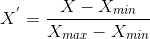This is how the formula for the process works where xmax and xmin are minimum and maximum values and here’s the code:

```# data normalization with sklearn
from sklearn.preprocessing import MinMaxScaler

# fit scaler on training data
norm = MinMaxScaler().fit(X_train)

# transform training data
X_train_norm = norm.transform(X_train)

# transform testing dataabs
X_test_norm = norm.transform(X_test)```

This is how the output would look like :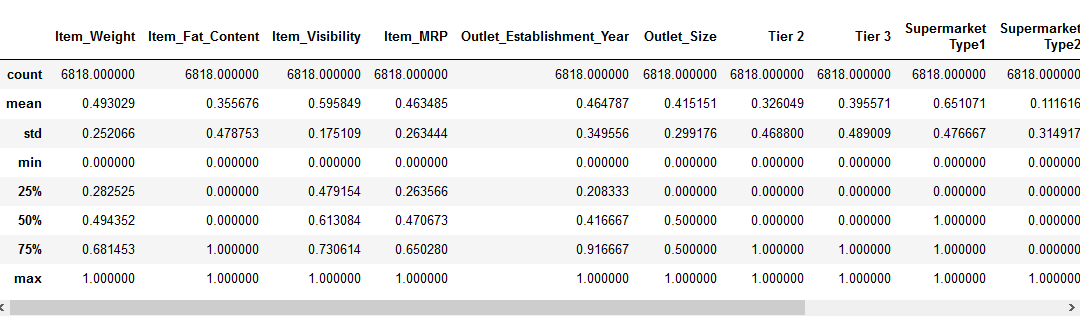It can be noticed that each and every value is between 0 and 1. Next comes standardization.

Standardization is centered around a mean which has a standard deviation. This is how the mean of all the attribute become zero and hence, the resultant of the whole distribution is now zero.

Here is the formula for this :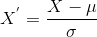Let’s take a look at the code :

```# data standardization with  sklearn
from sklearn.preprocessing import StandardScaler

# copy of datasets
X_train_stand = X_train.copy()
X_test_stand = X_test.copy()

# numerical features
num_cols = ['Item_Weight','Item_Visibility','Item_MRP','Outlet_Establishment_Year']

# apply standardization on numerical features
for i in num_cols:

# fit on training data column
scale = StandardScaler().fit(X_train_stand[[i]])

# transform the training data column
X_train_stand[i] = scale.transform(X_train_stand[[i]])

# transform the testing data column
X_test_stand[i] = scale.transform(X_test_stand[[i]])```

The look would look something like this :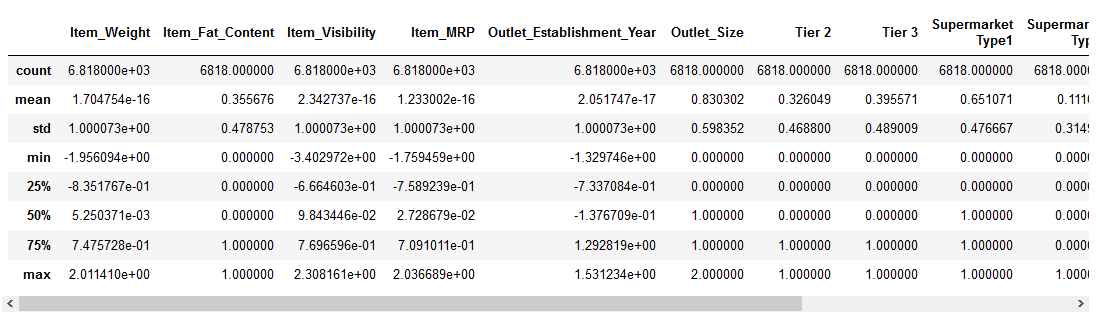## Through Our Eyes :

This is how web scraping can be useful in the process of data analysis by data preprocessing.

In the case, any user has found this difficult, we at Scrapingpass.com will be more than happy to assist you with any kind of web scraping related query.RocketSource

In the guide, we have carefully displayed the best and the most efficient methodology you can use to implement the concept of web scraping in the machine learning model.

While there are many guidelines that can direct any person towards the correct methodology but, this is the best available one. You can find everything you need to know about web scraping.